# R语言预测人口死亡率：用李·卡特（Lee-Carter）模型、非线性模型进行平滑估计

``````
D=DEATH\$Male
E=EXPO\$Male
A=as.numeric(as.character(DEATH\$Age))
Y=DEATH\$Year
I=(A<100)
base=data.frame(D=D,E=E,Y=Y,A=A)
subbase=base[I,]
subbase=subbase[!is.na(subbase\$A),]``````

​可以使用

``````
ylab="Years (1900-2005)",zlab="Mortality rate (log)")``````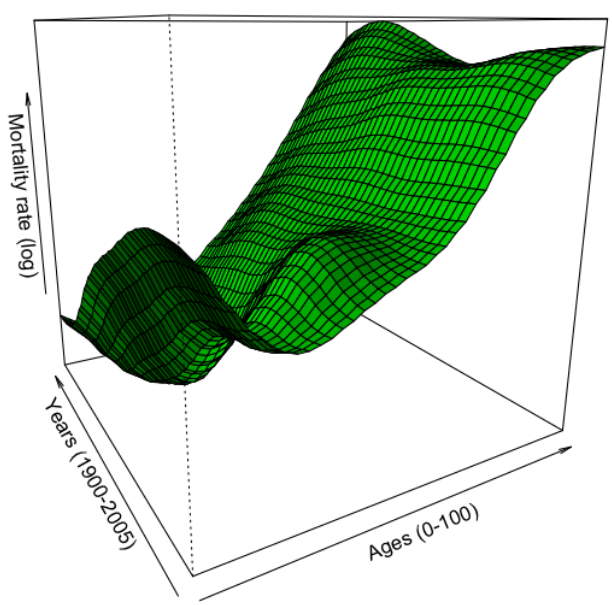``````predAx=function(a) mean(predict(regbsp,newdata=data.frame(A=a,
Y=seq(min(subbase\$Y),max(subbase\$Y)),E=1)))
plot(seq(0,99),Vectorize(predAx)(seq(0,99)),col="red",lwd=3,type="l")``````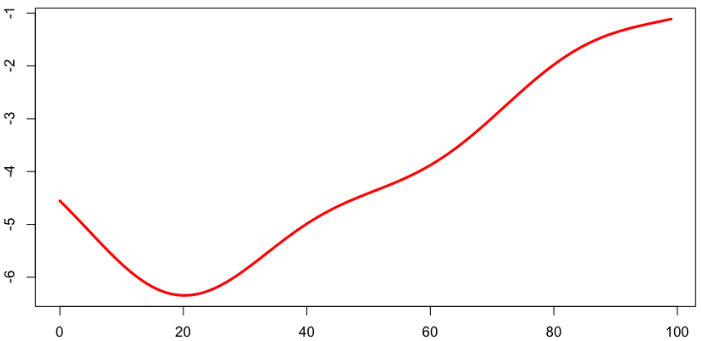``````persp(vZ,theta=-30,col="green",shade=TRUE,xlab="Ages (0-100)",
ylab="Years (1900-2005)",zlab="Mortality rate (log)")``````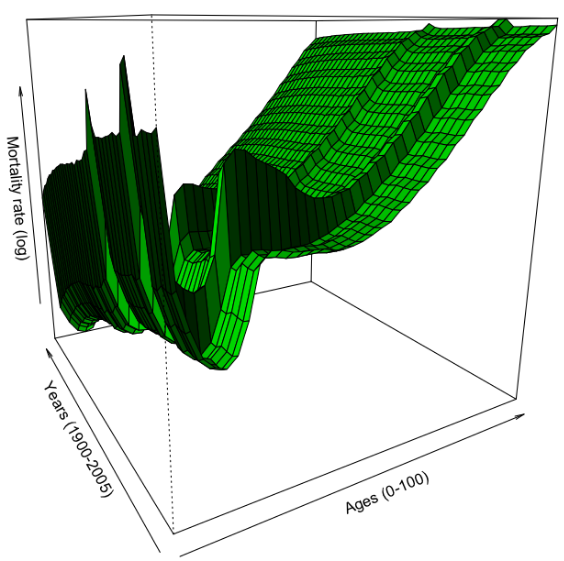``plot(seq(1,99),coefficients(regnp)[2:100],col="red",lwd=3,type="l")``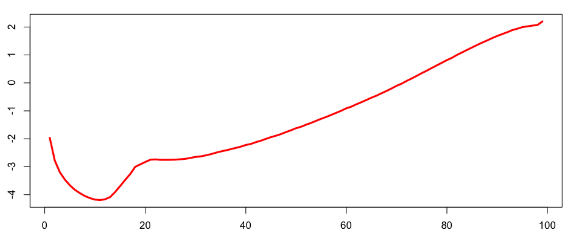# R语言Lee-Carter模型对年死亡率建模预测期望寿命

代码片段

``````
ylab="Years (1900-2005)",zlab="Mortality rate (log)")``````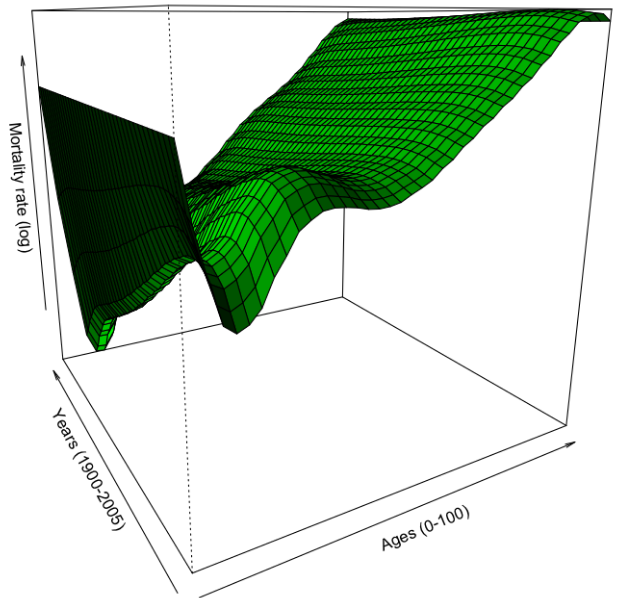### 随时关注您喜欢的主题

``````BpA=bs(seq(0,99),knots=knotsA,Boundary.knots=range(subbase\$A),degre=3)
Ax=BpA%*%coefficients(regsp)[2:8]
plot(seq(0,99),Ax,col="red",lwd=3,type="l")``````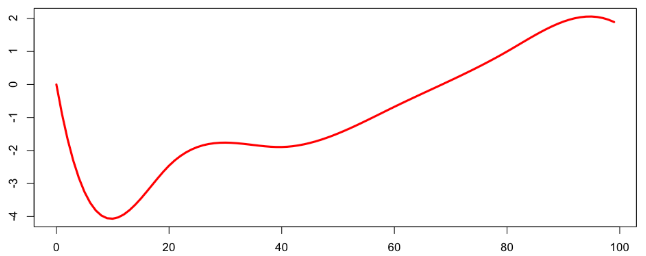``````persp(vZ,theta=-30,col="green",shade=TRUE,xlab="Ages (0-100)",
ylab="Years (1900-2005)",zlab="Mortality rate (log)")``````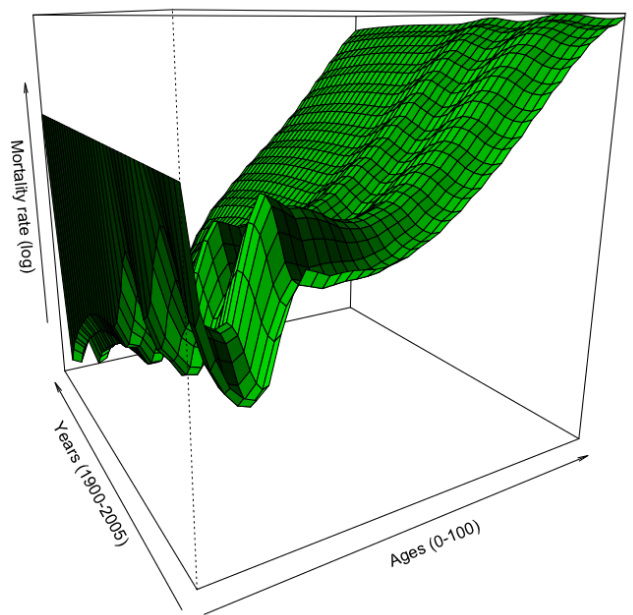Kaizong Ye拓端研究室（TRL）的研究员。

​非常感谢您阅读本文，如需帮助请联系我们！QQ在线咨询

15121130882

0571-63341498

## 关注有关新文章的微信公众号

This will close in 0 seconds